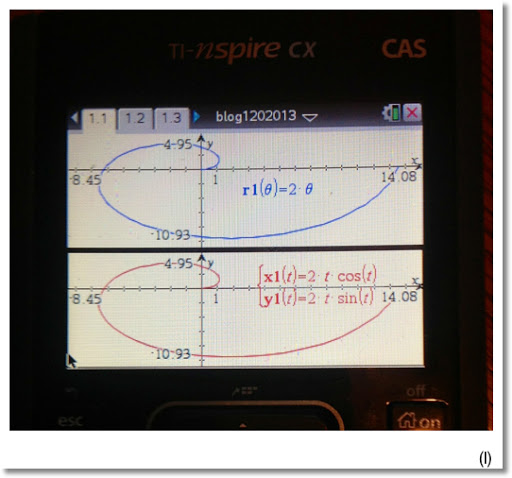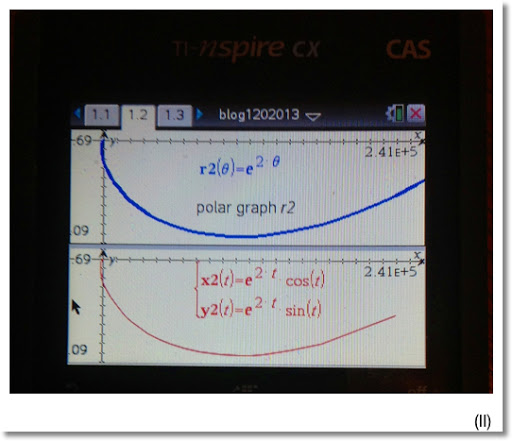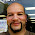## Sunday, January 20, 2013

### Converting Polar Equations to Parametric Equations

Good Sunday!

This blog will show how to transform polar equations, in the form of r(θ) to a pair of parametric equations, x(t) and y(t).

Tools We Need

x = r * cos θ
y = r * sin θ

Let θ = t.

For each example, we will change each polar equation and display a graph for each form. The polar form, colored blue, is on top; the parametric form, in red, is on the bottom. (A TI nSpire-CX is used for the pictures)

Example (I):

r = 2*θ

Then θ = r/2 and let θ = t.

x = r * cos t
x = 2 * t * cos(r/2)
x = 2 * t * cos((2*t)/2)
x = 2 * t * cos t

Similarly,

y = r * sin t
y = 2 * t * sin(r/2)
y = 2 * t * sin t

To summarize:
r = 2*θ

is equivalent to

x = 2 * t * cos t
y = 2 * t * sin tExample (II):

r = e^(2 * θ)

Then θ = 1/2 * ln r and let θ = t.

And...

x = r * cos t
x = e^(2*t) * cos(1/2 * ln r)
x = e^(2*t) * cos(1/2 * ln(e^(2*t)))
x = e^(2*t) * cos(1/2 * 2 * t)
x = e^(2*t) * cos t

y = r * sin t
y = e^(2*t) * sin(1/2 * ln r)
y = e^(2*t) * sin(1/2 * ln(e^(2*t)))
y = e^(2*t) * sin t

To summarize:
r = e^(2*θ)

is equivalent to:

x = e^(2*t) * cos t
y = e^(2*t) * sin tExample (III):

r = 2 cos θ

Then:

θ = arccos(r/2) = cos⁻¹(r/2)

Then, with θ=t...

x = r * cos t
x = 2 * cos t * cos(cos⁻¹(r/2))
x = 2 * cos t * cos(cos⁻¹(2*cos(t)/2))
x = 2 * cos t * cos t
x = 2 * cos² t

And... (some trigonometric identities are required)

y = r * sin t
y = 2 * cos t * sin(cos⁻¹(r/2))

Note: sin(cos⁻¹ x) = √(1 - x²)

y = 2 * cos t * √((1 - (r/2)²)
y = 2 * cos t * √(1 - r²/4)
y = 2 * cos t * √(1 - (4 cos² t)/4)
y = 2 * cos t * √(1 - cos² t)

Note: sin² x + cos² x = 1

y = 2 * cos t * sin t

Note: sin(2*x) = 2 * cos x * sin x

y = sin (2*t)

To Summarize:
r = 2 cos θ

is equivalent to:

x = 2 * cos² t
y = sin (2*t)Until next time, take care!

Eddie

This blog is property of Edward Shore. 2013

#### 5 comments:

1.thanks for share...

2.1.You are quite welcome, Naveen and city!

3.Hope this blog isn't dead!
Struggling with this:
How can I modify the parametric equation of an archimedean spiral so it can be rotated by X degrees? I really can't figure this out!

1.Didn't explain myself correctly:
I want to find the intersection between each layer of the spiral. I thought rotating it would do the trick but it's not the case! Using parametric equations:
-Normal archimedean spiral:
x=r*t*(cos(t))
y=r*t*(sin(t))
-Rotated archimedean spiral:
x=r*(cos(t)-t*sin(t))
y=r*(sin(t)+t*cos(t))

### HP Prime and HP 41C/DM 41L: Sum of Two Squares

HP Prime and HP 41C/DM 41L:  Sum of Two Squares  Introduction Given a positive integer n, can we find two non-negative integers x and y...